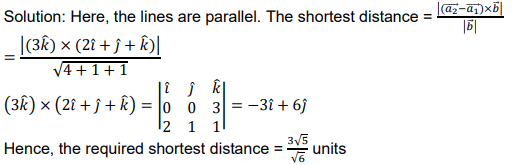# Find the shortest distance between the following lines: 𝑟⃗ = (𝑖̂+ 𝑗̂− 𝑘̂) + 𝑠(2𝑖̂+ 𝑗̂+ 𝑘̂) 𝑟⃗ = (𝑖̂+ 𝑗̂+ 2𝑘̂) + 𝑡(4𝑖̂+ 2𝑗̂+ 2𝑘̂)

CBSE Sample Question Paper, Class 12 Mathematics Term 2 Question - Find the shortest distance between the following lines: 𝑟⃗ = (𝑖̂+ 𝑗̂− 𝑘̂) + 𝑠(2𝑖̂+ 𝑗̂+ 𝑘̂) 𝑟⃗ = (𝑖̂+ 𝑗̂+ 2𝑘̂) + 𝑡(4𝑖̂+ 2𝑗̂+ 2𝑘̂)## Find the shortest distance between the following lines: 𝑟⃗ = (𝑖̂+ 𝑗̂− 𝑘̂) + 𝑠(2𝑖̂+ 𝑗̂+ 𝑘̂) 𝑟⃗ = (𝑖̂+ 𝑗̂+ 2𝑘̂) + 𝑡(4𝑖̂+ 2𝑗̂+ 2𝑘̂)

### Answer. Find the shortest distance between the following lines: 𝑟⃗ = (𝑖̂+ 𝑗̂− 𝑘̂) + 𝑠(2𝑖̂+ 𝑗̂+ 𝑘̂) 𝑟⃗ = (𝑖̂+ 𝑗̂+ 2𝑘̂) + 𝑡(4𝑖̂+ 2𝑗̂+ 2𝑘̂)